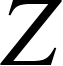## Voltage Drop

Last updated on 2020-02-27 4 mins. to read

Voltage drop is calculated in accordance with CENELEC technical report CLC/TR 50480 "Determination of cross-sectional area of conductors and selection of protective devices", dated February 2011.

The voltage drop (as a percentage) is given by:

$ΔU= b( Rcosφ+Xsinφ ) I b U o ⋅100$

where
ΔU         = cable voltage drop, %
Uo             = nominal  line to neutral voltage, V
R           = cable resistance, Ω
X           = cable reactance, Ω
Ib           = cable design current, A
b            = circuit factor (=2 for d.c. and single-phase, =1 for three-phase)

Note: within myCableEngineering, we use complex arithmetic and the above is evaluated as:

$ΔU= b( R+jX ) I b U o ⋅100$

Note: R and X are per line conductor.  For example, the resistance of a single-phase two core circuit (live and neutral) would be 2R (assuming the live and neutral circuits are of equal resistance).  For parallel cables, R and X are the per line resultant values. For example, nph cables in parallel, with the resistance of one line conductor is Rc0ph then the R = Rc0ph/nph.

The above equation), has the relative voltage drop expressed as a percentage of Uo.  Multiplying by Uo will give the actual voltage drop for d.c. and single-phase circuits and for three-phase circuits, this needs to be multiplied by √3.

Adjusting the CENELEC equation to take into account referring the voltage drop to line-line voltage for three-phase systems, setting R, X in ohms, and using complex forms gives:

ΔU, V/m ΔU, % per m
d.c.  systemsa.c.  systems, single-phasea.c systems, three-phase-  the resistance of a single conductor, Ω/m- the reaction of a single conductor,  Ω/m- the impedance of single conductor , Ω/m- cable design current, A- the nominal line to neutral/earth voltage (for single-phase a.c. or d.c.), V- the nominal line to line voltage (for three-phase systems), V

### BS 7671  Voltage Drop Tables

BS7671 "Requirements for Electrical Installations", the IET Wiring Regulations, Appendix 4 voltage drop tables, values are given in mV/A/m (or effectively mΩ).  However, these tables are related to the line to neutral voltage for single-phase circuits and line to line voltage for three-phase circuits.
To convert the three-phase table values so that they relate to the input resistance (or reactance) required by CENELEC 50480 it is necessary to divide the BS 7671 three-phase values by √3 (the square root of three).  No adjustment is required for single phase values.  Given values of voltage drop for three-phase balanced systems are related to the line voltage.
Note: the values in the tables are given at the maximum conductor operating temperature.  myCableEngineering uses circuit theory (see ) to calculate impedance and voltage drops are calculated using CENELEC 50480.  Our testing shows that the difference in resistance calculation by circuit theory and that given in BS 7671 is small.